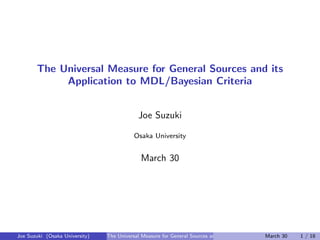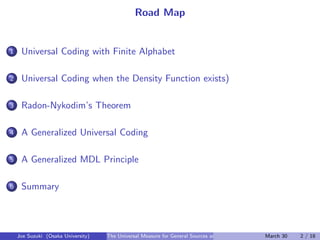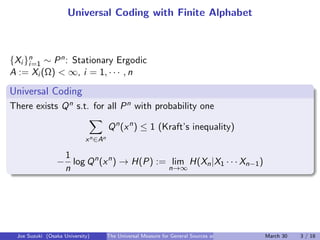Successfully reported this slideshow.

# The Universal Measure for General Sources and its Application to MDL/Bayesian Criteria

0

Share×
1 of 18
1 of 18

# The Universal Measure for General Sources and its Application to MDL/Bayesian Criteria

0

Share

Joe Suzuki, DCC 2011

Joe Suzuki, DCC 2011

## More Related Content

### Related Books

Free with a 14 day trial from Scribd

See all

### Related Audiobooks

Free with a 14 day trial from Scribd

See all

### The Universal Measure for General Sources and its Application to MDL/Bayesian Criteria

1. 1. The Universal Measure for General Sources and its Application to MDL/Bayesian Criteria Joe Suzuki Osaka University March 30 Joe Suzuki (Osaka University) The Universal Measure for General Sources and its Application to MDL/Bayesian CriteriaMarch 30 1 / 18
2. 2. Road Map ...1 Universal Coding with Finite Alphabet ...2 Universal Coding when the Density Function exists) ...3 Radon-Nykodim’s Theorem ...4 A Generalized Universal Coding ...5 A Generalized MDL Principle ...6 Summary Joe Suzuki (Osaka University) The Universal Measure for General Sources and its Application to MDL/Bayesian CriteriaMarch 30 2 / 18
3. 3. Universal Coding with Finite Alphabet {Xi }n i=1 ∼ Pn: Stationary Ergodic A := Xi (Ω) < ∞, i = 1, · · · , n . Universal Coding .. ...... There exists Qn s.t. for all Pn with probability one ∑ xn∈An Qn (xn ) ≤ 1 (Kraft’s inequality) − 1 n log Qn (xn ) → H(P) := lim n→∞ H(Xn|X1 · · · Xn−1) Joe Suzuki (Osaka University) The Universal Measure for General Sources and its Application to MDL/Bayesian CriteriaMarch 30 3 / 18
4. 4. Universal Coding with Finite Alphabet (cont’d) Shannon-McMillan-Breiman: with probability one − 1 n log Pn (xn ) → H(P) . We wish to generalize that .. ...... there exists Qn s.t. for all Pn with probability one 1 n log Pn(xn) Qn(xn) → 0 Joe Suzuki (Osaka University) The Universal Measure for General Sources and its Application to MDL/Bayesian CriteriaMarch 30 4 / 18
5. 5. Universal Coding when the Density Function exists {Xi }n i=1 ∼ f n: Stationary Ergodic {Ak}∞ k=1 Ak is a Partion of Xi (Ω) Ak+1 is a Reﬁnment of Ak with A0 := {Xi (Ω)} ex. Xi (Ω) = [0, 1) A1 = {[0, 1/2), [1/2, 1)} A2 = {[0, 1/4), [1/4, 1/2), [1/2, 3/4), [3/4, 1)} . . . Ak = {[0, 2−(k−1)), [2−(k−1), 2 · 2−(k−1)), · · · , [(2k−1 − 1)2−(k−1), 1)} . . . Joe Suzuki (Osaka University) The Universal Measure for General Sources and its Application to MDL/Bayesian CriteriaMarch 30 5 / 18
6. 6. Universal Coding when the Density Function exists (cont’d) sk : Rn → An k (Projection) Pk: the Probability of sk(Xn) λn: Lebesgue Measure . For each k, there exists universal Qk .. ...... fk(xn ) := Pk(sk(xn)) λn(sk(xn)) , gk(xn ) := Qk(sk(xn)) λn(sk(xn)) 1 n log Pk(sk(xn)) Qk(sk(xn)) → 0 {ωk}∞ k=1: ∑ ωk = 1, ωk > 0 g(xn ) := ∞∑ k=1 ωkgk(xn ) Joe Suzuki (Osaka University) The Universal Measure for General Sources and its Application to MDL/Bayesian CriteriaMarch 30 6 / 18
7. 7. Universal Coding when the Density Function exists (cont’d) h(f ) := lim n→∞ ∫ −f (xn ) log f (xn|x1, · · · , xn−1)dxn . We wish to generalize .. ...... If we choose {Ak}∞ k=1 s.t. h(fk) → h(f )(k → ∞), there exists gn ( ∫ ∞ −∞ gn(xn)dxn ≤ 1) s.t. for all f n, with probability one 1 n log f n(xn) gn(xn) → 0 B. Ryabko. IEEE Trans. on Information Theory, VOL. 55, NO. 9, 2009. Joe Suzuki (Osaka University) The Universal Measure for General Sources and its Application to MDL/Bayesian CriteriaMarch 30 7 / 18
8. 8. What if there exists no Density Function ex. ∫ ∞ 0 h(x)dx = 1 and FX (x) =    0 x < −1, 1 2 , −1 ≤ x < 0∫ x 0 1 2 h(t)dt, 0 ≤ x =⇒ there exists no fX s.t. FX (x) = ∫ x −∞ fX (t)dt By what are P(xn) Q(xn) , f (xn) g(xn) expressed in the general setting of {Xi }n i=1? Joe Suzuki (Osaka University) The Universal Measure for General Sources and its Application to MDL/Bayesian CriteriaMarch 30 8 / 18
9. 9. Random Variables (Ω, F, µ): Probability Space B: the Borel set in R . Xis a Random Variable .. ...... F-measurable X : Ω → R, i.e. D ∈ B =⇒ {ω ∈ Ω|X(ω) ∈ D} ∈ F Finite Sources Continuous Sources with Density Functions Continuous Sources without Density Functions Joe Suzuki (Osaka University) The Universal Measure for General Sources and its Application to MDL/Bayesian CriteriaMarch 30 9 / 18
10. 10. Radon-Nykodim’s Theorem . µ is Absolutely Continiuous w.r.t. ν (µ << ν) .. ...... for each A ∈ F ν(A) = 0 =⇒ µ(A) = 0 . Radon-Nykodim derivative dµ dν.. ...... µ << ν ⇐⇒ there exists F-measureble g : Ω → R s.t. for each A ∈ F µ(A) = ∫ A g(ω)dν(ω) λ: Lebesgue measure on R . Density function fX exists .. ......⇐⇒ µ << λ for FX (x) := µ(ω ∈ Ω|X(ω) ≤ x) Joe Suzuki (Osaka University) The Universal Measure for General Sources and its Application to MDL/Bayesian CriteriaMarch 30 10 / 18
11. 11. Kullback-Leibler Information . Kullback-Leibler Information .. ...... When µ << ν D(µ||ν) := ∫ dµ log dµ dν Finite Source: P, Q =⇒ dµ dν (xn ) = P(xn) Q(xn) D(µn ||νn ) = ∑ xn∈An P(xn ) log Pn(xn) Qn(xn) Continuous Source with Density Function: f , g =⇒ dµ dν (xn ) = f (xn) g(xn) D(µn ||νn ) = ∫ f n (xn ) log f n(xn) gn(xn) dxn Joe Suzuki (Osaka University) The Universal Measure for General Sources and its Application to MDL/Bayesian CriteriaMarch 30 11 / 18
12. 12. Construction of Measure νn Qn k (an) , an ∈ An k ηn: µn << ηn (ηn = λn =⇒ Ryabko)   For each (D1, · · · , Dn) ∈ Bn νn k (D1, · · · , Dn) := ∑ a1,··· ,an∈Ak ηn(a1 ∩ D1, · · · , an ∩ Dn) ηn(a1, · · · , an) Qn k (a1, · · · , an) . ( ⇐⇒ dνn k dηn := Qn k (a1, · · · , an) ηn(a1, · · · , an) )   {ωi }∞ k=0: ∞∑ k=0 ωk = 1, ωk > 0 νn (D1, · · · , Dn) := ∞∑ k=0 ωkνn k (D1, · · · , Dn) Joe Suzuki (Osaka University) The Universal Measure for General Sources and its Application to MDL/Bayesian CriteriaMarch 30 12 / 18
13. 13. A Generalized Universal Coding µn k(D1, · · · , Dn) := ∑ a1,··· ,an∈Ak ηn(a1 ∩ D1, · · · , an ∩ Dn) ηn(a1, · · · , an) Pn k (a1, · · · , an) . D(µ||ν) := lim n→∞ ∫ dµ(xn ) log dµ dν (xn|x1, · · · , xn−1) . Theorem .. ...... If we choose {Ak}∞ k=1 s.t. D(µk||η) = D(µ||η) (k → ∞), there exists νn ( ∫ xn∈Xn(Ω) dνn(xn) ≤ 1) s.t. for all µn, with probability one 1 n log dµn dνn (x1, · · · , xn) → 0 Joe Suzuki (Osaka University) The Universal Measure for General Sources and its Application to MDL/Bayesian CriteriaMarch 30 13 / 18
14. 14. An Example not realized by the existing Universal Coding X(Ω) := N = {1, 2, · · · }, η(j) = 1 j − 1 j + 1 , j ∈ N A1 := {{1}, N − {1}} A2 := {{1}, {2}, N − {1, 2}} · · · Ak := {{1}, {2}, · · · , {k}, N − {1, · · · , k}} · · · Qn k (sk(xn)): 1 n log Pn k (sk(xn)) Qn k (sk(xn)) → 0 , n → ∞ The Probability of j ∈ N − {1, · · · , k} is to be proporional to η(j) = 1 j − 1 j + 1 Joe Suzuki (Osaka University) The Universal Measure for General Sources and its Application to MDL/Bayesian CriteriaMarch 30 14 / 18
15. 15. Case Study 1: Markov Order Estmation . The Markov Order .. ...... For each n = 1, 2, · · · , the minimum s s.t. {Xj }∞ j=n ⊥⊥ {Xj }n−s−1 j=1 |{Xj }n−1 j=n−s {Xi }n i=1 ∼ Pn[s]: Markov with order s π[s]: the a Prior Probability of Order s   If Xi (Ω) = A < ∞, ...1 for each s = 0, 1, · · · , we estimate Qn[s]: ▶ ∑ xn∈An Qn [s](xn ) ≤ 1 ▶ 1 n log Pn [s](xn ) Qn[s](xn) → 0 ...2 Given a Sequence xn, we choose s maximizing π[s]Qn[s](xn) (minimizing ⇐⇒ − log π[s] − log Qn[s](xn)) Joe Suzuki (Osaka University) The Universal Measure for General Sources and its Application to MDL/Bayesian CriteriaMarch 30 15 / 18
16. 16. Case Study 1: Markov Order Estmation (cont’d) In general, in the neighborhood of xn, maximizing π[s]νn[s](∆xn) (⇐⇒ minimizing − log π[s] − log νn[s](∆xn))   . Decision Rule .. ...... ...1 Construct νn[s] for each s = 0, 1, · · · , ▶ ∑ xn∈An νn [s](xn ) ≤ 1 ▶ 1 n log dµn [s] dνn[s] (xn ) → 0 ...2 Given Sequence xn, π[s] π[s′] · dνn[s] dνn[s′] (xn ) > 1 ⇐⇒ s is better than s′ The Ratios of Probabilities and Density Functions are Radon-Nykodim Derivative in the general setting. Joe Suzuki (Osaka University) The Universal Measure for General Sources and its Application to MDL/Bayesian CriteriaMarch 30 16 / 18
17. 17. Case Study 2: Discrete and Continuous Features are mixed in Pattern Recognition S∗: Finite Set {Xk}k∈S∗ , Y : Random Variables   xn := {(xi,k)k∈S∗ }n i=1, yn := {yi }n i=1: Examples Finite Case: choose S ⊆ S∗ maximizing R(xn , yn , S) := π(S) Qn [xn , yn |S] Qn [xn |S] General Case: choose S ⊆ S∗ maximizing R(xn, ∆yn, S) := π(S) dνn [∆xn , ∆yn |S] dνn [∆xn |S] xn dR(xn, ∆yn, S) dR(xn, ∆yn, S′) yn > 1 ⇐⇒ S is better than S′ . Conditional Probability of Y given X .. ...... µ(Y ∈ D|X = x) := f (Y ∈ D|x) = dµ(X ∈ ∆x, Y ∈ D) dµ(X ∈ ∆x) Joe Suzuki (Osaka University) The Universal Measure for General Sources and its Application to MDL/Bayesian CriteriaMarch 30 17 / 18
18. 18. Contribution . New Theory .. ...... Universal Coding without assuming Discrete and Continuous Sources The MDL Principle without assuming Discrete and Continuous Sources . Applications .. ...... Previously, discrete and Continuous cases were separated Markov Order Estimation (Continuous Data Sequence) Feature Selection (Discrete and continuous features are mixed) BN Structure Estimation (Discrete and continuous rvs are mixed) . Feature Work .. ...... Computation Applications Joe Suzuki (Osaka University) The Universal Measure for General Sources and its Application to MDL/Bayesian CriteriaMarch 30 18 / 18